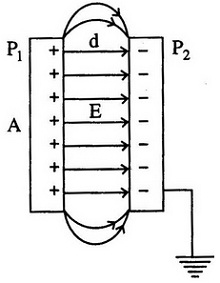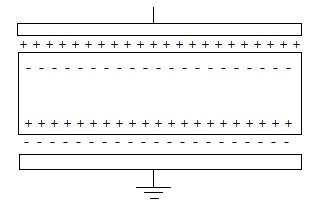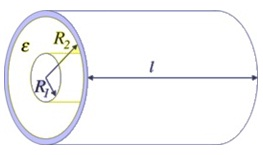#### Capacitor, Physics tutorial

Capacitance:

A capacitor or a condenser is the electronic device for storing electrical energy by permitting charges to build up on metal plates. This electrical energy is recovered if these charges are permitted to move away from these plates into the circuit of which the capacitor makes a part. Any device that can store charges is a capacitor. For instance, an insulated conducting spherical shell of radius 'R' can store charges; therefore it can be employed as a condenser. The potential of the outer surface of the shell is represented by:

Φ = Q/4πεoR

having infinity as zero potential. Rather than infinity we can regard the ground (earth) as zero potential. Then the capacitance of this shell (with respect to ground) is as:

C = Q/Φ = 4πεoR Coulomb/Volt

The unit of capacitance 'C' in SI system is the farad.

Parallel Plate Capacitor or Condenser:This is the simplest and most generally employed form of a condenser. The parallel plate condenser comprises of two rectangular or circular sheets (or plates) of a metal arranged parallel to one other, separated by a distance 'd'.  The value of 'd' is generally extremely small and an insulating material is generally inserted among the two sheets. A charge Q (positive) put on the upper plate distributes uniformly on this plate to make it an equipotential surface. The lower plate is revealed grounded. The lower plate is thus at ground potential (zero potential). As electrostatic induction an equivalent amount of negative charge comes out on the upper side of the lower plate. This induced negative charge pulls up nearly all the positive charge positioned on the upper plate to the lower side of the upper plate. Therefore the electric field now gets imprisoned to the space among the two plates: the positive charge acting as sources and the negative charge as sink (that is, the lines of force originate on the positive charges and end on negative charges).

The induced negative charge is equivalent to the amount of positive charge due to the reason of the zero field requirements within the material of the conducting sheets. Alongside, both the metal sheets are equipotential surfaces. The lines of force field lines are normal to such sheets apart from at edges. As all the field lines originate from the upper plate and end on the lower plate, the value of the electric field, 'E' is uniform in the space among the plates apart from the edge. The edge effects are minor if the area of the plates, 'A', is large as compared to 'd'. As 'E' is uniform, the potential difference between the upper and the lower plates is represented by:

E = σ/εo

V = Ed

Placing the value of 'E'

V = (σ/εo) x d

However σ = Q/A

V = Qd/A.εo

A.εoV = Qd

We are familiar that the electric charge stored on any one of plate of capacitor is: Q = CV

Placing the value of 'Q' in above

oV = CVd

Cd = Aεo

C = Aεo/d

Energy Stored in a Capacitor:

The work done 'W' in assembling a charge 'Q' by adding infinitesimal increments of charge is represented by:

W = 1/2 QΦ

Here Φ is the final potential of the charged body. In case of a capacitor of capacitance 'C', this work done in putting a charge 'Q' on the capacitor should as well be given by similar expression, that is,

W = 1/2 QΦ

As C = Q/Φ

W = 1/2 CΦ2 = Q2/2C Joules

The work done is stored in the electric field as the potential energy.

Parallel Plate Capacitor with Dielectrics:If a dielectric slab is inserted between the parallel plates of a condenser the capacity rises. The polarized dielectric slab ABCD decreases the electric field 'E' within the dielectric by a factor (1/εr) here εr is the relative permittivity.

DS = σ S

As the bound surface charges don't contribute to this flux and

D = εoεr E

For an isotropic uniformly polarized dielectric. Therefore the field:

E = σ/εoεr

The potential difference between the plates is equivalent to Ed, where 'd' is now the thickness of the slab filling the whole space between the plates.

The capacitance now becomes:

C = Q/Φ = σA/Ed = εoεrA/Ed

The value of capacitance 'C' raises by the factor εr that is the relative permittivity of the dielectric material. From the above equation we observe that the capacitance of a parallel plate capacitor rises with the increase in surface area 'A' of the plates and as well by the decrease of the distance separating the plates.

The result of introducing a dielectric in between the plates raises the capacitance (that is, εr > 1). Therefore the inclusion of a dielectric lets the capacitor to hold more charges at a given potential difference between the plates.

We can rewrite the equation as:

C = εoA/(d/εr)

Voltage Rating of a Capacitor:

The capacitors are manufactured and designed to operate at a certain maximum voltage that is based on the distance between the plates of the capacitor. When the voltage is surpassed, the electrons jump across the space between the plates and this can outcome in permanent damage to the capacitor. The maximum safe voltage is termed as the working voltage. The capacity and the working voltage (WV) is marked on the capacitor in case of bigger capacitors and pointed by the color code (that is, similar to that of resistance) the case of capacitors having low values of capacitance.

Capacitance of a Cylindrical Capacitor:

A capacitor is build up of two concentric cylindrical shell or wires separated to one other through a dielectric in between them as shown in the figure below is termed as the Cylindrical Capacitor.Assume that the radius of the inner cylinder is R1 and that of the outer cylinder is R2. And assume that the relative permittivity of the medium in between the two cylinders be εr

Suppose the charge per meter length of the cable on the outer surface of the inner cylinder be +Q coulomb and on the inner surface of the outer cylinder be -Q coulombs. For all practical rationale, the charge of +Q coulombs/m on the surface of the core can be supposed to be positioned at its axis. The outer cylinder is earthed.

Now, the surface area of the coaxial cylinder of radius 'x' meters and length of one meter is 2πxm2

Therefore, the electrical field intensity at a point 'x' meters away from the center of the inner cylinder is represented as:

Ex = Q/2πεoεrx

Ex = (Q/2πεoεr) (1/x) V/m

Therefore, the potential difference widened between the two plates or cylinders in the parallel plate capacitor (V) is:

V = R1R2 -Edx

V = R1R2 -Qdx/2πεoεrx

V = - Q/2πεoεr R1R2 (dx/x)

V = - Q/2πεoεr loge R1 - loge R2

V = - Q/2πεoεr loge (R1/R2)

Q/V = 2πεoεr/loge (R2/R1)

Or, the capacitance 'C' in the case is:

C = 2πεoεr/loge (R2/R1) F/m

Therefore, the capacitance of a cylindrical capacitor with 'L' meter length or height and R1, R2 is the radius of inner and outer cylinder correspondingly is:

C = [2πεoεrL/loge (R2/R1)] F

Capacitors in Series and Parallel:

Series Capacitors Equation:

If adding altogether Capacitors in Series, the reciprocal (1/C) of the individual capacitors are all added altogether (just similar to resistors in parallel) rather than the capacitance's themselves. Then the net value for capacitors in series equivalents the reciprocal of the sum of the reciprocals of the individual capacitances.

1/CT = 1/C1 + 1/C2 + 1/C3 +.....

Parallel Capacitors Equation:

We can state the net or total circuit capacitance, CT as being the sums of all the individual capacitances add altogether giving us the generalized equation of:

CT = C1 + C2 + C3 +.....

Practical Capacitors:

Capacitors might be widely categorized into two groups that are, fixed and variable capacitors. They might be further categorized according to their construction and use. The given are some of the types of the capacitor.

1) Fixed Capacitors:

The capacitor whose value is fixed throughout the manufacturing procedure and can't be later modifies is termed as the fixed capacitor.

a) Paper Capacitor: A paper capacitor is made up of two thin tinfoil sheets that are separated through thin waxed and oiled paper or plastic, the sandwich of thin foils and paper or plastic is then rolled to a cylindrical shape that is then enclosed in plastic capsule. The two tin foils of the capacitors are joined to two external leads.

The capacitance of paper capacitor can range in between 0.001-2.000 Micro farad and the voltage rating can be as high as around 2000 Voltage.

b) Mica Capacitor: To build a mica capacitor a few thin plate of metal are sandwiched separated via thin sheets of mica, the alternate plate are then joined to form two terminals that are joined to two leads and the entire assembly is surrounded in plastic of Bakelite capsule. Mica capacitors encompass small capacitance in the range of around 50 to 500 picofarads; however encompass relatively high working voltage of up to 500 voltages.

c) Ceramic Capacitor: To build a Ceramic capacitor a hollow tubular or plate such as ceramic material like titanium dioxide and barium titanate is taken that is then coated by means of a deposit of silver compound on both inner and external surface of the ceramic, the two silver coating acts as two plates and the ceramic material acts as the dielectric. The two surfaces are then joined to leads and the entire assembly is then encapsulated in the moisture proof coating.

d) Electrolytic Capacitor: This kind of capacitor is a capacitor which uses an electrolyte as negative plate. Electrolyte capacitors have positive and negative plate and comprise of the given materials:

A positive plate builds up of aluminum

A very thin insulating film of aluminum oxide as dielectric that is electrochemically deposited in the surface of positive aluminum plate itself.

An electrolyte of borax or carbon salt that is absorbed through a absorbent gauze which is positioned in contact by the dielectric and joined to an external aluminum lead acting as a  negative lead.

2) Variable Capacitors:

A variable capacitor is the capacitor whose capacitance can be differed as needed generally by rotating a shaft joined to the capacitor.

A normal variable capacitor comprises of a Multi Plate parallel plate capacitor where one set of plates is stationary that is termed stator whereas the other sets of plates is rotatable and is joined to a shaft and by rotating the shaft the two sets of plates can be configured to come over each other in a requisite amount that finds out the capacitance of the capacitor, if the sets of plates are fully over one other the capacitance is highest.

If two or more capacitors are joined to a common shaft the configuration is termed as a ganged capacitor; ganged capacitors is generally employed having short wave radio equipments.

The variable capacitors can as well be made by two small flexible metal plates separated by air, mica or ceramic slab as dielectric. The spacing between the plates can be modified by a means of screw; these types of variable capacitors are generally small in size and are termed as trimmer. They are generally employed in circuits for small adjustments or fine tuning.

Tutorsglobe: A way to secure high grade in your curriculum (Online Tutoring)

Expand your confidence, grow study skills and improve your grades.

Since 2009, Tutorsglobe has proactively helped millions of students to get better grades in school, college or university and score well in competitive tests with live, one-on-one online tutoring.

Using an advanced developed tutoring system providing little or no wait time, the students are connected on-demand with a tutor at www.tutorsglobe.com. Students work one-on-one, in real-time with a tutor, communicating and studying using a virtual whiteboard technology.  Scientific and mathematical notation, symbols, geometric figures, graphing and freehand drawing can be rendered quickly and easily in the advanced whiteboard.

Free to know our price and packages for online physics tutoring. Chat with us or submit request at info@tutorsglobe.com